Function Repository Resource:

# RungeKuttaOrderConditions

List equations for Runge-Kutta methods

Contributed by: Wolfram Research
 ResourceFunction["RungeKuttaOrderConditions"][p,s] gives a list of the order conditions that any s-stage Runge-Kutta method of order p must satisfy. ResourceFunction["RungeKuttaOrderConditions"][p] gives the order conditions using stage-independent tensor notation.

## Details and Options

The order conditions are grouped by order.
The following options can be given:
 "ButcherRowSum" False whether to include row-sum conditions for ci "ButcherSimplify" False whether to use Butcher's assumptions "ContinuousExtension" False whether to generate conditions for continuous extensions "RungeKuttaMethod" "Implicit" type of Runge-Kutta method
If the option "ButcherSimplify"True is selected, the number of stages must be a positive integer.
The s-stage Runge-Kutta method to advance from x to x+h is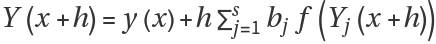where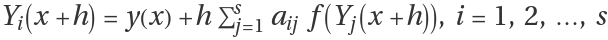, aij and bj are unknown coefficients and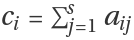, i=1,2,,s.
Valid settings for "RungeKuttaMethod" are "DiagonallyImplicit", "Explicit" and "Implicit".

## Examples

### Basic Examples (1)

Give the order conditions for any third-order method:

 In:=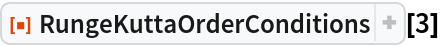Out=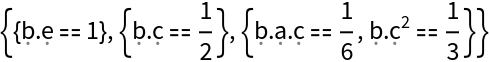### Scope (4)

The number of order conditions goes up greatly from order to order:

 In:=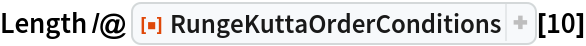Out=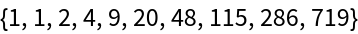The order conditions that must be satisfied by any second-order, three-stage Runge-Kutta method:

 In:=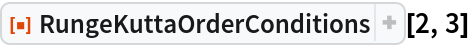Out=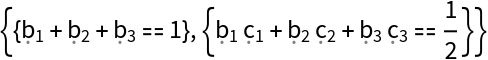The order conditions that must be satisfied by any second-order, s-stage method:

 In:=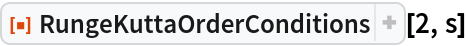Out=Replacing s with 3 gives the same result as RungeKuttaOrderConditions[2,3]:

 In:=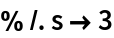Out=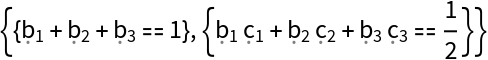Not all sets of border conditions can be satisfied. A fourth-order, one-stage Runge-Kutta method would need more stages to be solvable:

 In:=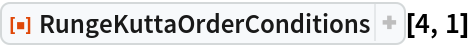Out=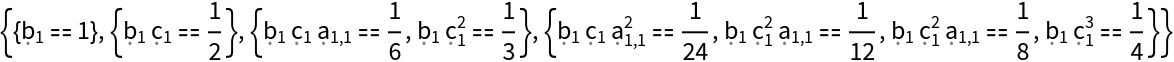In:=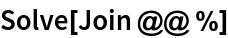Out=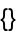### Options (3)

#### ButcherRowSum (1)

The conditions that must be satisfied by any first-order, three-stage Runge-Kutta method, including the row-sum conditions:

 In:=Out=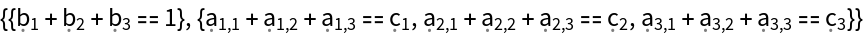#### RungeKuttaMethod (2)

The order conditions that must be satisfied by any third-order, two-stage, diagonally implicit Runge-Kutta method:

 In:=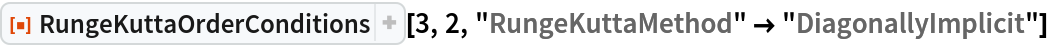Out=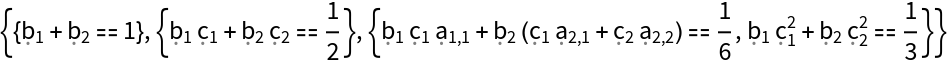The contradiction in the order conditions for any third-order, two-stage, explicit Runge-Kutta method indicates that no such method is possible:

 In:=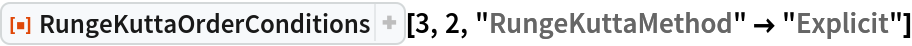Out=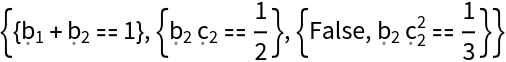### Properties and Relations (1)

Th resource function ButcherPrincipalError can be used to determine the principal error coefficients:

 In:=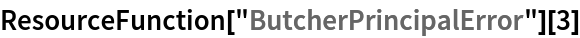Out=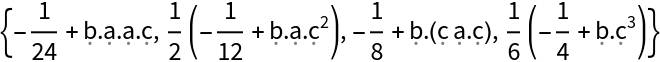## Version History

• 1.0.0 – 06 November 2019